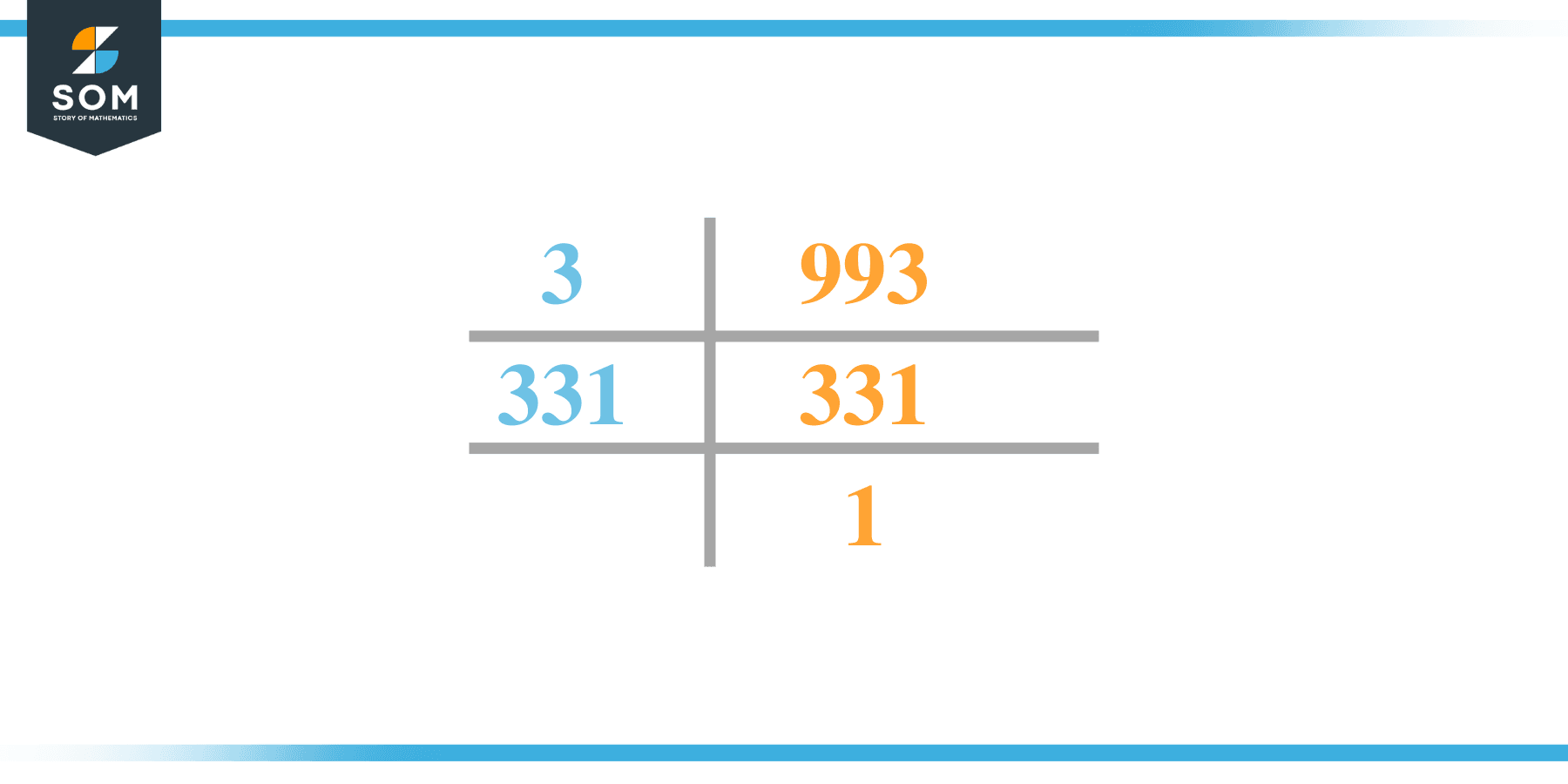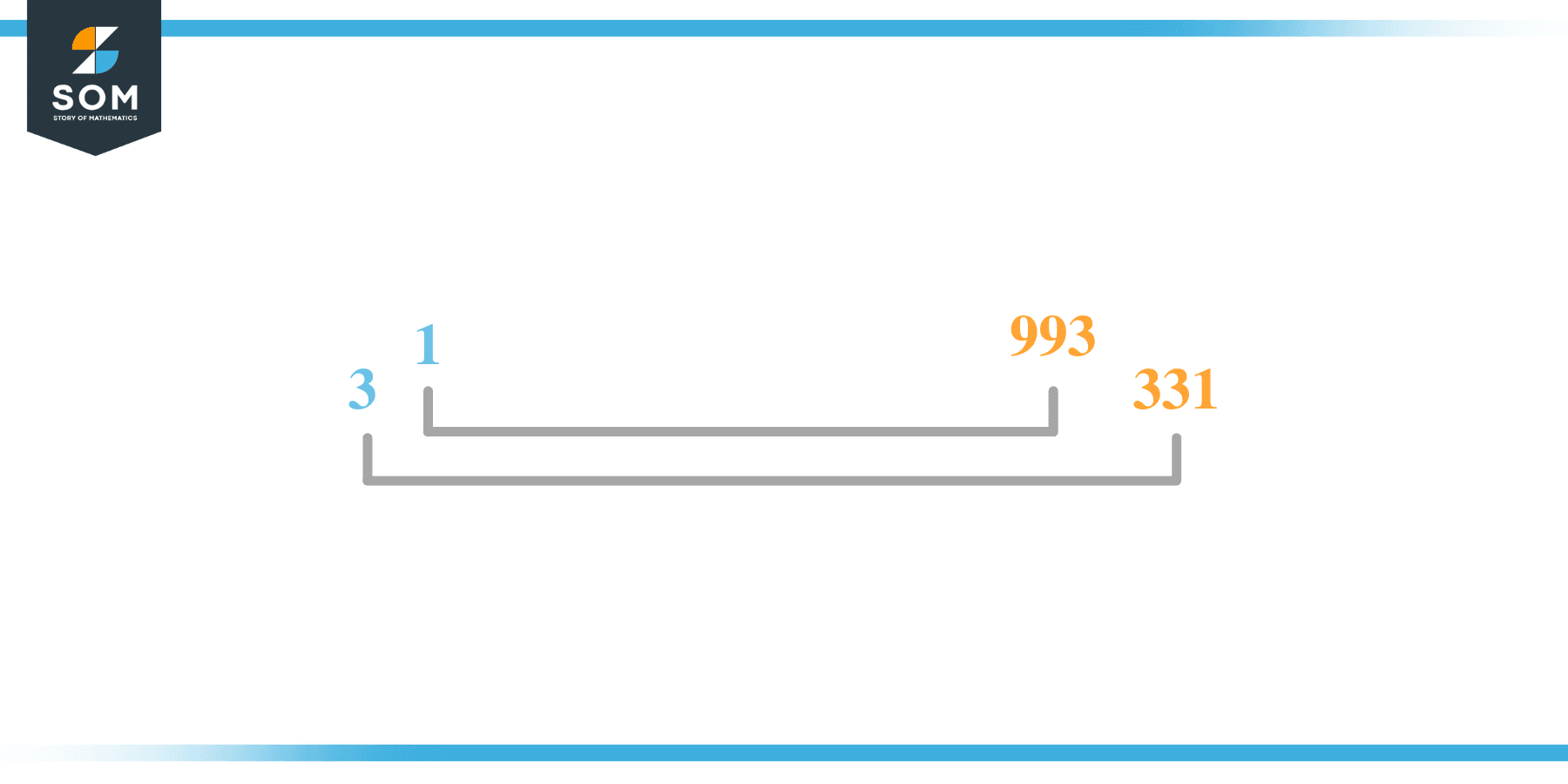# Factors of 993: Prime Factorization, Methods, and Examples

The factors of 993 are listing the integers that divide 993 entirely without leaving any remainder behind. There are four factors of 993 in total. The given number’s factors can be positive or negative.### Factors of 993

Here are the factors of number 993.

Factors of 993: 1, 3, 331, and 993.

### Negative Factors of 993

The negative factors of 993 are similar to their positive aspects, just with a negative sign.

Negative Factors of 993: –1, -3, -331, and -993

### Prime Factorization of 993

The prime factorization of 993 is the way of expressing its prime factors in the product form.

Prime Factorization: 3 x 331

In this article, we will learn about the factors of 993 and how to find them using various techniques such as upside-down division, prime factorization, and factor tree.

## What Are the Factors of 993?

The factors of 993 are 1, 3, 331, and 993. These numbers are the factors as they do not leave any remainder when divided by 993.

The factors of 993 are classified as prime numbers and composite numbers. The prime factors of the number 993 can be determined using the prime factorization technique.

## How To Find the Factors of 993?

You can find the factors of 993 by using the rules of divisibility. The divisibility rule states that any number, when divided by any other natural number, is said to be divisible by the number if the quotient is the whole number and the resulting remainder is zero.

To find the factors of 993, create a list containing the numbers that are exactly divisible by 993 with zero remainders. One important thing to note is that 1 and 993 are the 993’s factors, as every natural number has one, and the number itself as its factor.

One is also called the universal factor of every number. The factors of 993 are determined as follows:

$\dfrac{993}{1} = 993$

$\dfrac{993}{3} = 331$

Therefore, 1, 3, 331, and 993 are the factors of 993.

### Total Number of Factors of 993

For 993, there are four positive factors and four negative ones. So in total, there are eight factors out of 993.

To find the total number of factors of the given number, follow the procedure mentioned below:

1. Find the factorization/prime factorization of the given number.
2. Demonstrate the prime factorization of the number in the exponent form.
3. Add 1 to each of the exponents of the prime factor.
4. Now, multiply the resulting exponents together. This obtained product is equivalent to the total number of factors of the given number.

By following this procedure, the total number of factors of 993 is given as follows:

Factorization of 993 is 1 x 3 x 331.

The exponent of 1, 3, and 331 is 1.

Adding 1 to each and multiplying them together results in 993.

Therefore, the total number of factors of 993 is 8. Four are positive, and four factors are negative.

### Important Notes

Here are some essential points that must be considered while finding the factors of any given number:

• The factor of any given number must be a whole number.
• The number factors cannot be in the form of decimals or fractions.
• Factors can be positive as well as negative.
• Negative factors are the additive inverse of the positive factors of a given number.
• The factor of a number cannot be greater than that number.
• Every even number has 2 as its prime factor, the smallest prime factor.

## Factors of 993 by Prime FactorizationThe number 993 is a composite number. Prime factorization is a valuable technique for finding the number’s prime factors and expressing the number as the product of its prime factors.

Before finding the factors of 993 using prime factorization, let us find out what prime factors are. Prime factors are the factors of any given number that are only divisible by 1 and themselves.

To start the prime factorization of 993, start dividing by its most minor prime factor. First, determine that the given number is either even or odd. If it is an even number, then 2 will be the smallest prime factor.

Continue splitting the quotient obtained until 1 is received as the quotient. The prime factorization of 993 can be expressed as:

993 = 3 x 331

## Factors of 993 in PairsThe factor pairs are the duplet of numbers that, when multiplied together, result in the factorized number. Factor pairs can be more than one depending on the total number of factors given.

For 993, the factor pairs can be found as:

1 x 993 = 933

3 x 331 = 993

The possible factor pairs of 993 are given as (1, 993) and (3, 331).

All these numbers in pairs, when multiplied, give 993 as the product.

The negative factor pairs of 993 are given as:

-1 x -993 = 993

-3 x -331 = 993

It is important to note that in negative factor pairs, the minus sign has been multiplied by the minus sign, due to which the resulting product is the original positive number. Therefore, –1, -3, -331, and -993 are called negative factors of 993.

The list of all the factors of 993, including positive as well as negative numbers, is given below.

Factor list of 993: 1, -1, 3, -3, 331, -331, 993, and -993

## Factors of 993 Solved Examples

To better understand the concept of factors, let’s solve some examples.

### Example 1

How many factors of 993 are there?

### Solution

The total number of Factors of 993 is 8.

Factors of 993 are 1, 3, 331, and 993.

### Example 2

Find the factors of 993 using prime factorization.

### Solution

The prime factorization of 993 is given as:

993 $\div$ 3 = 331

331 $\div$ 331 = 1

So the prime factorization of 993 can be written as:

3 x 331 = 993